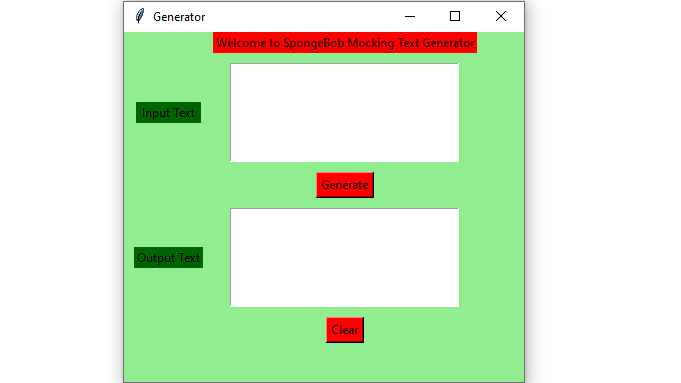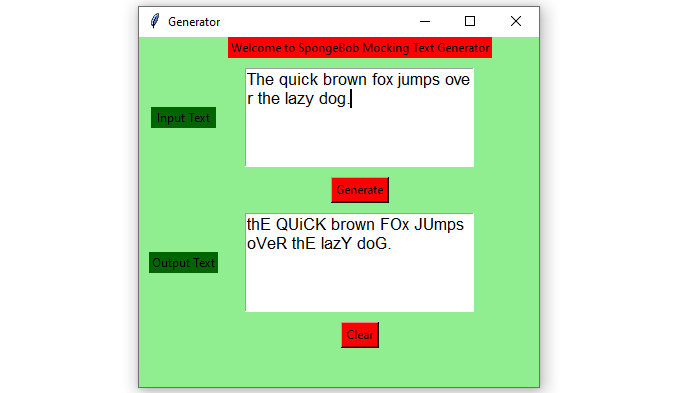# Python – SpongeBob Mocking Text Generator GUI using Tkinter

Prerequisites : Introduction to tkinter | SpongeBob Mocking Text Generator

Python offers multiple options for developing a GUI (Graphical User Interface). Out of all the GUI methods, Tkinter is the most commonly used method. Python with tkinter outputs the fastest and easiest way to create GUI applications. In this article, we will learn how to create a SpongeBob Mocking Text Generator GUI application using Tkinter, with a step-by-step guide.

To create a tkinter :

• Importing the module – tkinter
• Create the main window (container)
• Add any number of widgets to the main window.
• Apply the event Trigger on the widgets.

Let’s create a GUI based SpongeBob Mocking Text Generator application that can generate SpongeBob Text from the input given by user.

Below is the implementation :

 `# import all functions from the tkinter   ` `from` `tkinter ``import` `*` ` `  `# import the random library  ` `import` `random ` ` `  `# Function to clear both the text areas ` `def` `clearAll() : ` `    ``# whole content of text area  is deleted  ` `    ``text1_field.delete(``1.0``, END) ` `    ``text2_field.delete(``1.0``, END) ` ` `  `# Function to Generate SpongeBob Mocking Text ` `def` `generate() : ` ` `  `    ``# get a whole input content from text box ` `    ``# ignoring \n from the text box content ` `    ``input_text ``=` `text1_field.get(``"1.0"``, ``"end"``)[:``-``1``] ` `     `  `    ``# variable declaration for the output text  ` `    ``output_text ``=` `""  ` `       `  `    ``# check the cases for every individual character  ` `    ``for` `char ``in` `input_text:  ` `           `  `        ``# check if the character is an alphabet  ` `        ``if` `char.isalpha():  ` `               `  `            ``# convert to upper case  ` `            ``if` `random.random() > ``0.5``:  ` `                ``output_text ``+``=` `char.upper()  ` `                   `  `            ``# convert to lower case  ` `            ``else``:  ` `                ``output_text ``+``=` `char.lower()  ` `           `  `        ``# if character is not and alphabet  ` `        ``# add it as it is  ` `        ``else``:  ` `            ``output_text ``+``=` `char  ` `   `  `    ``text2_field.insert(``'end -1 chars'``, output_text) ` ` `  ` `  `# Driver code  ` `if` `__name__ ``=``=` `"__main__"` `:  ` ` `  `    ``# Create a GUI window  ` `    ``root ``=` `Tk() ` ` `  `    ``# Set the background colour of GUI window   ` `    ``root.configure(background ``=` `'light green'``)   ` `     `  `    ``# Set the configuration of GUI window (WidthxHeight)  ` `    ``root.geometry(``"400x350"``)   ` ` `  `    ``# set the name of tkinter GUI window   ` `    ``root.title(``"Generator"``) ` `     `  `    ``# Create Welcome to SpongeBob Mocking Text Generator label   ` `    ``headlabel ``=` `Label(root, text ``=` `'Welcome to SpongeBob Mocking Text Generator'``,   ` `                      ``fg ``=` `'black'``, bg ``=` `"red"``)   ` `   `  `    ``# Create a "Input Text " label   ` `    ``label1 ``=` `Label(root, text ``=` `" Input Text "``,  ` `                 ``fg ``=` `'black'``, bg ``=` `'dark green'``)  ` `       `  `    ``# Create a "Output Text " label   ` `    ``label2 ``=` `Label(root, text ``=` `"Output Text"``,   ` `                   ``fg ``=` `'black'``, bg ``=` `'dark green'``)   ` `     `  `   `  `    ``# grid method is used for placing   ` `    ``# the widgets at respective positions   ` `    ``# in table like structure . ` `    ``headlabel.grid(row ``=` `0``, column ``=` `1``) ` `     `  `    ``# padx keyword argument used to set paading along x-axis . ` `    ``label1.grid(row ``=` `1``, column ``=` `0``, padx ``=` `10``)   ` `    ``label2.grid(row ``=` `3``, column ``=` `0``, padx ``=` `10``)  ` ` `  `       `  `    ``# Create a text area box   ` `    ``# for filling or typing the information.   ` `    ``text1_field ``=` `Text(root, height ``=` `5``, width ``=` `25``, font ``=` `"lucida 13"``)  ` `    ``text2_field ``=` `Text(root, height ``=` `5``, width ``=` `25``, font ``=` `"lucida 13"``) ` `        `  `    ``# padx keyword argument used to set paading along x-axis . ` `    ``# pady keyword argument used to set paading along y-axis .   ` `    ``text1_field.grid(row ``=` `1``, column ``=` `1``, padx ``=` `10``, pady ``=` `10``)   ` `    ``text2_field.grid(row ``=` `3``, column ``=` `1``, padx ``=` `10``, pady ``=` `10``)  ` ` `  `       `  `    ``# Create a Generate Button and attached   ` `    ``# with generate function   ` `    ``button1 ``=` `Button(root, text ``=` `"Generate"``, bg ``=` `"red"``, fg ``=` `"black"``,  ` `                                ``command ``=` `generate)  ` `       `  `    ``button1.grid(row ``=` `2``, column ``=` `1``)  ` `   `  `    ``# Create a Clear Button and attached   ` `    ``# with clearAll function   ` `    ``button2 ``=` `Button(root, text ``=` `"Clear"``, bg ``=` `"red"``,   ` `                     ``fg ``=` `"black"``, command ``=` `clearAll) ` `     `  `    ``button2.grid(row ``=` `4``, column ``=` `1``)  ` `     `  `    ``# Start the GUI   ` `    ``root.mainloop()   `

Output :My Personal Notes arrow_drop_upCheck out this Author's contributed articles.

If you like GeeksforGeeks and would like to contribute, you can also write an article using contribute.geeksforgeeks.org or mail your article to contribute@geeksforgeeks.org. See your article appearing on the GeeksforGeeks main page and help other Geeks.

Please Improve this article if you find anything incorrect by clicking on the "Improve Article" button below.

Article Tags :

Be the First to upvote.

Please write to us at contribute@geeksforgeeks.org to report any issue with the above content.